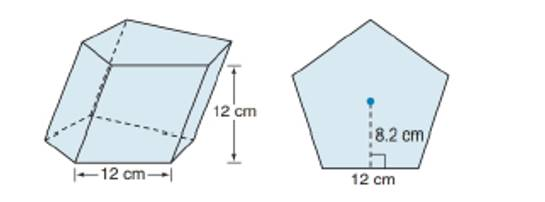Chapter 9.1, Problem 45EElementary Geometry For College St...

7th Edition
Alexander + 2 others
ISBN: 9781337614085

Solutions

Chapter
SectionElementary Geometry For College St...

7th Edition
Alexander + 2 others
ISBN: 9781337614085
Textbook Problem

For Exercise 45 to 47, consider the oblique regular pentagonal prism shown. Each side of the base measures 12 cm, and the altitude measures 12 cm.Find the Internal area of the prism.(HINT: Each lateral face is a parallelogram.)To determine

To find:

The lateral area of the prism.

Explanation

The lateral area of any solid is the area of all the number of lateral sides that it have, excluding the area of the top and the bottom of the solid.

The lateral area (L) of any solid prison with the perimeter of the base of the prism as (P) and altitude ‘h’ is given by the formula

L=Ph.

Here, P is the perimeter of any one of the base of the prism and which is given by the sum of all the sides of the base.

Calculation:

Given,

The measure of the base edge of the regular pentagonal = 12 cm.

The altitude of the measures = 12 cm.

The lateral area of any prism of altitude ‘h’ and of base perimeter P is given by,

L=hP

Here, we have

h=12 cm

Still sussing out bartleby?

Check out a sample textbook solution.

See a sample solution

The Solution to Your Study Problems

Bartleby provides explanations to thousands of textbook problems written by our experts, many with advanced degrees!

Get Started

In Exercises 63-68, rationalize the denominator. 66. 5x23x

Applied Calculus for the Managerial, Life, and Social Sciences: A Brief Approach

Write each expression in terms of i. 8

Trigonometry (MindTap Course List)

In Exercises 724, compute the indicated products. [0.10.90.20.8][1.20.40.52.1]

Finite Mathematics for the Managerial, Life, and Social Sciences